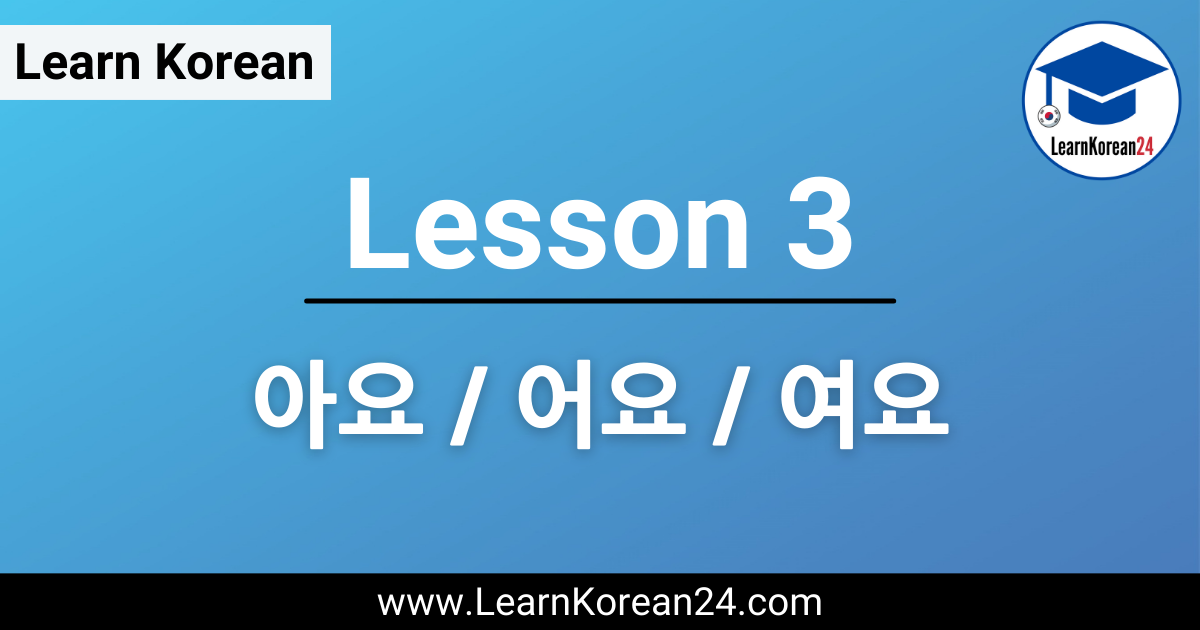# Lesson 3: 아/어/여요In this lesson, you will learn how to take the dictionary form of a verb and change it into the present tense by adding 아요, 어요, or 여요. We’ll also introduce the concept of Korean conjugation and you’ll learn the steps you need to take to conjugate a verb and make the present tense.

## Lesson 3:아/어/여요

When you look up Korean verbs in a dictionary you will find that they are written in the infinitive form and each verb ends in 다 [da]. For example, ‘to eat’ in Korean is 먹다 [meok-da]. When you want to use a Korean verb in a sentence, you must first remove 다 so you are left with the ‘verb stem’. So, the verb stem of 먹다 [meok-da] is 먹 [meok].

Once you have the verb stem, you can then attach different endings to the verb to express different tenses and grammatical functions. Changing a verb or adjective in this way is known as conjugation. In this lesson, we will just be focusing on the present tense conjugation 아/어/여요.

*Please note that in spoken Korean, the present tense form can also mean the present progressive tense (e.g. eating, studying, cleaning, etc.). There is a specific way to make the present progressive form, but Koreans often just use the present tense form instead.

### 아요 [a-yo]

If the last vowel in the verb stem is either ㅏ or ㅗ then we attach 아요 to the verb stem to get the present tense. Let’s look at an example:

살다 [sal-da] = to live (dictionary form of verb)
[sal] = live (verb stem)
살아요 [sa-ra-yo] = live (present tense)

As you can see in the example above, after removing 다 from 살다 you are left with the verb stem 살. The final vowel in 살 is ㅏ, and so we attach 아요 to make 살아요.

### 어요 [eo-yo]

If the last vowel in the verb stem is NOT ㅏ or ㅗ then we attach 어요 to the verb stem to get the present tense. Let’s look at an example:

먹다 [meok-da] = to eat (dictionary form of verb)
[meok] = eat (verb stem)
먹어요 [meo-geo-yo] = eat (present tense)

As you can see in the example above, after removing 다 from 먹다 you are left with the verb stem 먹. The final vowel in 먹 is ㅓ, and so we attach 어요 to make 먹어요.

### 여요 [yeo-yo]

If a verb ends in 하다, then we add 여요 to change that verb into the present tense. 하다 [ha-da] means ‘to do’ and many Korean verbs are simply a noun plus 하다. These so-called ‘하다 verbs’ are the easiest to learn because they are all conjugated in the same way.

Like other verbs, first, we must remove 다 from 하다 so we are left with the verb stem 하. Then we attach 여요 to make 해요 [hae-yo]. (Originally 하+여요 became 하여요 but over time this became 해요. All you need to remember is that 하다 verbs in the present tense are 해요.) Here are some more examples:

공부하다 [gong-bu-ha-da] = to study (dictionary form)
공부해요 [gong-bu-hae-yo] = study (present tense)
청소하다 [cheong-so-ha-da] = to clean (dictionary form)
청소해요 [cheong-so-hae-yo] = clean (present tense)

In this lesson, we covered the basic conjugation rules to use 아요, 어요, or 여요 to change verbs into the present tense. If you want a more detailed look into the present tense and to try some practice exercises, you can check our guide to the Korean present tense. Or if you’re ready to try and change verbs into the present tense on your own, then you can check out this list of 100 common Korean verbs.